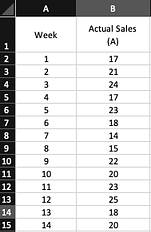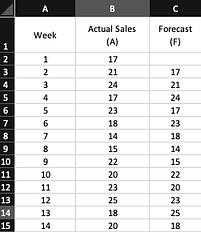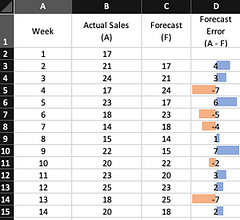# Basics of Forecast AccuracyIt is near impossible to calculate the accurate forecast despite the availability of multiple forecasting methods. And it is recommended that we should run various forecast models and compare them to find out which model is giving us a more accurate forecast. Let's dive into basics Forecast accuracy

It is near impossible to calculate the accurate forecast despite the availability of multiple forecasting methods. And it is recommended that we should run various forecast models and compare them to find out which model is giving us a more accurate forecast.

## Why do we need forecast accuracy?

For measuring accuracy, we compare the existing data with the data obtained by running the prediction model for existing periods. The difference between the actual and predicted value is also known as forecast error. Lesser the forecast error, the more accurate our model is.

By selecting the method that is most accurate for the data already known, it increases the probability of accurate future values.

## Measures of forecast accuracy

There are several measures to measure forecast accuracy:

· Mean Forecast Error (MFE)

· Mean Absolute Error (MAE) or Mean Absolute Deviation (MAD)

· Root Mean Square Error (RMSE)

· Mean Absolute Percentage Error (MAPE)

Let us consider the following table for this example.The table shows the weekly sales volume of a store. The store manager is not savvy with forecasting models, so he considered the current week’s sales as a forecast for next week. This methodology is also known as the _naïve forecasting method _due to the nature of simplicity.## Calculating Forecast Error

The difference between the actual value and the forecasted value is known as forecast error. So, in this example, forecast error for week 2 is

Forecast Error (week 2) = 21–17 = 4

A positive value of forecast error signifies that the model has underestimated the actual value of the period. A negative value of forecast error signifies that the model has overestimated the actual value of the period.

The following table calculates the forecast error for the rest of the weeks:## Create Forecast Using Excel 2016/2019

Data Science for Business Users. This tutorial was created to democratize data science for business users (i.e., minimize usage of advanced mathematics topics) and alleviate personal frustration we have experienced on following tutorials and struggling to apply that same tutorial for our needs

## Microsoft Excel Tutorial - Objects In Ms Excel- Excel Worksheet Objects

In this MS Excel tutorials, video we are going to see the differnce between tables and cell ranges in MS Excel. Also we are going to see that how can we create a Table from data given to us and what all changes we can apply to our tables.

## Microsoft Excel Tutorial - Slicers on Charts in MS Excel.

In this MS Excel tutorials, video we are going to apply the slicers in charts in MS Excel. However, there is no direct method of doing so, that is why we are going to use a simple trick over here. Also we are going to see that how can we connect same slicer with multiple charts in MS Excel. ​

## Excel VBA Tutorial for Beginners 19 - VBA Insert Cells in MS Excel

In this MS Excel VBA video, we are going to see the usage of the Insert Cells feature of MS Excel. Using this feature we can insert cells at some specified locations easily. In this video we will see the Overview of formulas in Excel.

## Excel VBA Tutorial for Beginners 11 - Excel VBA PasteSpecial Method

In this Excel VBA video, we are going to look at the usage of the Paste Special function in Excel using the VB code. Paste Special function helps us to use the paste function in different formats such as Pasting Cell Width, Pasting Text only, Pasting Cell Formats.